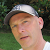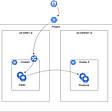# GKE’s “cluster-ipv4-cidr” flag

## Example #1: GKE cluster IP address space must not be already allocated on the GCE network

`\$CLUSTER=my-cluster\$NETWORK=my-network\$PROJECT=my-project\$REGION=us=west1\$SUBNET=my-subnet\$ZONE=us-west1-cgcloud compute networks create \${NETWORK} \--project=\${PROJECT} \--mode=customgcloud compute networks subnets create \${SUBNET} \--project=\${PROJECT} \--network=\${NETWORK} \--region=\${REGION} \--range=10.0.0.0/9`
`gcloud container clusters create \${CLUSTER} \--project=\${PROJECT} \--zone=\${ZONE} \--network=\${NETWORK} \--subnetwork=\${SUBNET} \--cluster-ipv4-cidr=10.1.0.0/19`
`ERROR:Requested CIDR 10.1.0.0/19 for containers is not available in network “\${NETWORK}” for cluster.`

## Example #2: How to determine the GKE cluster IP address space

`gcloud container clusters create \${CLUSTER} \--project=\${PROJECT} \--zone=\${ZONE} \--network=\${NETWORK} \--subnetwork=\${SUBNET} \--cluster-ipv4-cidr=10.128.0.0/19`
`gcloud compute instances list \--project=\${PROJECT}NAME           ZONE        INTERNAL_IP    STATUS84cdad0f-0t68  us-west1-c  10.0.0.2       RUNNING84cdad0f-t9hb  us-west1-c  10.0.0.3       RUNNING`
`kubectl describe node/84cdad0f-0t68Name:  84cdad0f-0t68Addresses:  InternalIP: 10.0.0.2  Hostname: 84cdad0f-0t68PodCIDR: 10.128.1.0/24`
`gcloud container clusters describe \${CLUSTER} \--project=\${PROJECT} \--zone=\${ZONE}clusterIpv4Cidr: 10.128.0.0/19servicesIpv4Cidr: 10.128.16.0/20status: RUNNINGsubnetwork: us-westzone: us-west1-b`

## Math Test #1: How many Nodes can this cluster support?

`gcloud container clusters resize \${CLUSTER} \--project=\${PROJECT} \--zone=\${ZONE} \--size=16`
`gcloud container clusters describe \${CLUSTER} \--project=\${PROJECT} \--zone=\${ZONE} \--format="json" \| jq '.currentNodeCount'16`
`gcloud container clusters resize \${CLUSTER} \--project=\${PROJECT} \--zone=\${ZONE} \--size=17`
`gcloud container clusters describe \${CLUSTER} \--project=\${PROJECT} \--zone=\${ZONE} \--format="json" \| jq '.currentNodeCount'16`
`gcloud container clusters describe \${CLUSTER} \--project=\${PROJECT} \--zone=\${ZONE} \--format="value(currentNodeCount)"`

by the author.

## More from Daz Wilkin

Love podcasts or audiobooks? Learn on the go with our new app.## Comparing External Secrets Operator with Secret Storage CSI as Kubernetes External Secrets is…## GKE Ingress redirect http to https## Security Implications of Cloud Computing## Service to Service call pattern — Using Anthos Service Mesh